# How to Get filename in Excel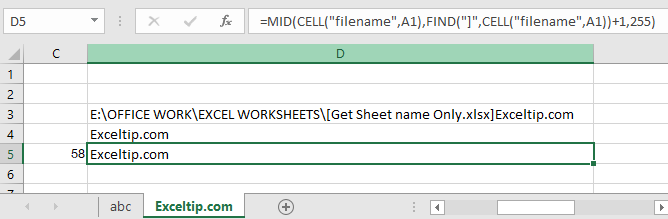In this article, we will learn How to Get filename in Excel.

Scenario:

Working with excel file information using excel formula is easy, convenient and common use. For example extracting the file name in excel

Formula to get file name

Cell function in Excel gets you the information regarding worksheets like col, contents, filename, ..etc.

Formula Syntax

 =CELL("filename",A1)

“filename” : gets the full name of the sheet of the reference cell

A1 : Sheet’s  cell reference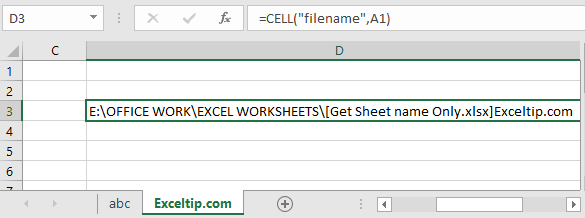But we need to extract just the sheet name. Basically the last name.

As you can see the sheet name starts after ] (closed big bracket sign). For that we just need its position in the text and then we will extract the sheet name.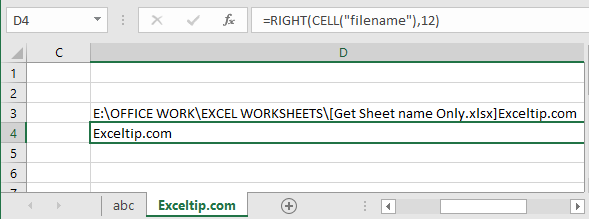Here I counted the characters from the last of the result which was 12. But we cannot do this every time.

So for extracting the sheet name from the full name, we need to find the position of the ] (closed big bracket sign)

Use the formula to find ]

 =FIND("]",CELL("filename",A1))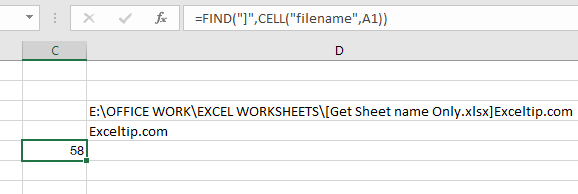58 is the position of ] .

Now we our formula is completed now

 =MID(CELL("filename",A1),FIND("]",CELL("filename",A1))+1,255)

Explanation:

CELL("filename",A1) : Gets you the full name of the worksheet

FIND("]",CELL("filename",A1))+1 : this function gets you the position of ] and add +1 because we need the position of the first char of only the sheet name.

255 : Max word limit of sheet name by Excel.

MID : MID function extracts the specific substring from the text using the position from start to end.Here we extracted the filename using the formula.

Now we have one more formula, instead of using MID function. Use the RIGHT function.

RIGHT function extracts the specific substring from the last of the text.

Use the formula:

 =RIGHT(CELL("filename",A2),LEN(CELL("filename",A2))-FIND("]", CELL("filename",A2)))

Explanation:

FIND("]", CELL("filename",A2)) : Extracts the position of sheet name char.

LEN(CELL("filename",A2)) : gets the length of the text(filename)

RIGHT : RIGHT function extracts the string from the text using its position.As you can see, this function works fine too. Yesss...Now we have 2 different formula with logic used to extract only the sheet name. Learn How to get only the sheet name using VBA in Excel here.

VBA example

We have data in three sheets and we want to consolidate data from all of them to the main sheet. Data from each sheet should have a sheet name at the end.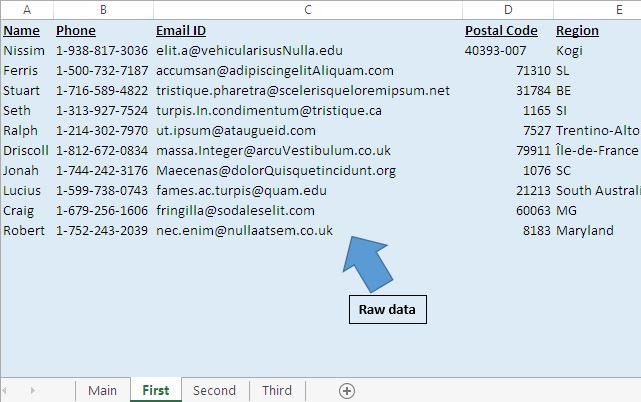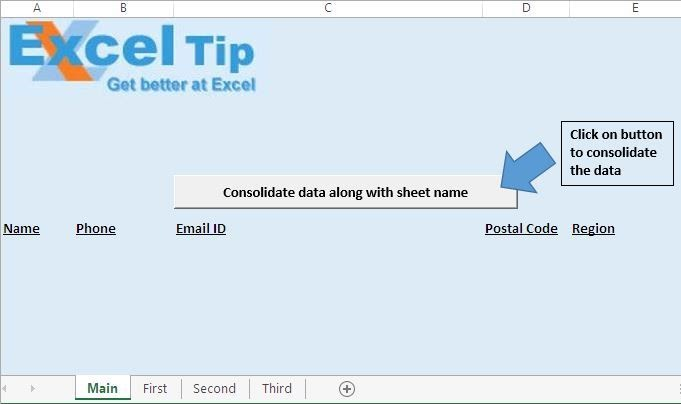On clicking the “Consolidate data along with sheet name” button, data will be consolidated on the main sheet.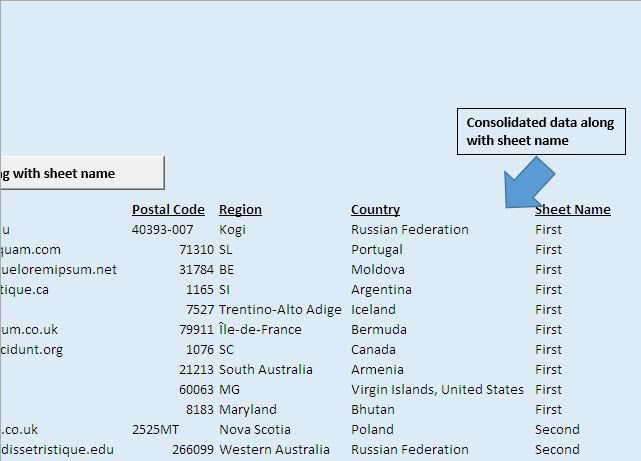Code explanation

SheetCount = Application.Worksheets.Count

The above code is used to get the count of worksheet within the workbook.

LastRow = ActiveCell.SpecialCells(xlLastCell).Row

The above code is used to get the row number of the last cell.

Range("A2:F" & LastRow).Select

The above code is used to select all the data starting from cell A2.

Selection.Copy

The above code is used to copy the selected data.

Range(Selection, Cells(LastRow, 7)).Value = Sheets(Counter).Name

The above code is used to assign the sheet name to the selected cell.

```Option Explicit

Sub ConsolidateDataWithSheetName()

'Declaring variables
Dim Counter As Integer
Dim SheetCount As Integer
Dim LastRow As Long

Application.ScreenUpdating = False

'Getting the count of worksheets in the workbook
SheetCount = Application.Worksheets.Count

For Counter = 2 To SheetCount

Sheets(Counter).Activate

Range("A2").Select

'Getting the row number of the last cell
LastRow = ActiveCell.SpecialCells(xlLastCell).Row

'Selecting all the data in the workbook
Range("A2:F" & LastRow).Select

'Copying the selected data
Selection.Copy

Sheets("Main").Activate

Range("A2").Select
LastRow = ActiveCell.SpecialCells(xlLastCell).Row
LastRow = LastRow + 1

'Pasting the copied data to last row on the Main sheet
Cells(LastRow, 1).Select
ActiveSheet.Paste

Cells(LastRow, 7).Select
LastRow = ActiveCell.SpecialCells(xlLastCell).Row

'Adding the sheet name along with the data
Range(Selection, Cells(LastRow, 7)).Value = Sheets(Counter).Name

Next

End Sub

```

Here are all the observational notes using the formula in Excel
Notes :

Hope this article about How to Get filename from path in Excel is explanatory. Find more articles on calculating values and related Excel formulas here. If you liked our blogs, share it with your friends on Facebook. And also you can follow us on Twitter and Facebook. We would love to hear from you, do let us know how we can improve, complement or innovate our work and make it better for you. Write to us at info@exceltip.com.

Related Articles :

Delete drop down list in Excel : The dropdown list is used to restrict the user to input data and gives the option to select from the list. We need to delete or remove the Dropdown list as the user will be able to input any data instead of choosing from a list.

How do I Insert a Checkmark in Excel 2016 : To insert a checkmark in Excel Cell we use the symbols in Excel. Set the fonts to wingdings and use the formula Char(252) to get the symbol of a check mark.

How to disable Scroll Lock in Excel : Arrow keys in excel that move sheet cell up, down, Left & Right. But this feature is only applicable when Scroll Lock in Excel is disabled. Scroll Lock in Excel is used to scroll up, down, left & right your worksheet not the cell. So this article will help you how to check scroll lock status and how to disable it?

How to Delete only Filtered Rows without the Hidden Rows in Excel : Many of you are asking how to delete the selected rows without disturbing the other rows. We will use the Find & Select option in Excel.

Popular Articles :